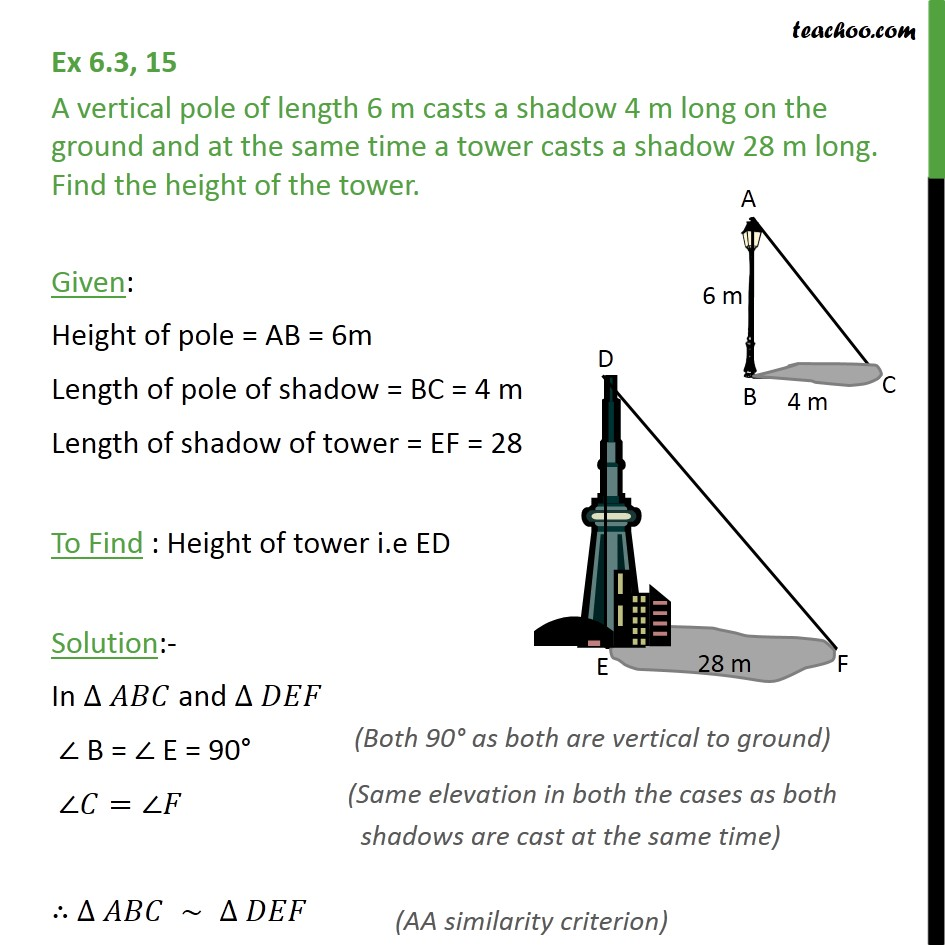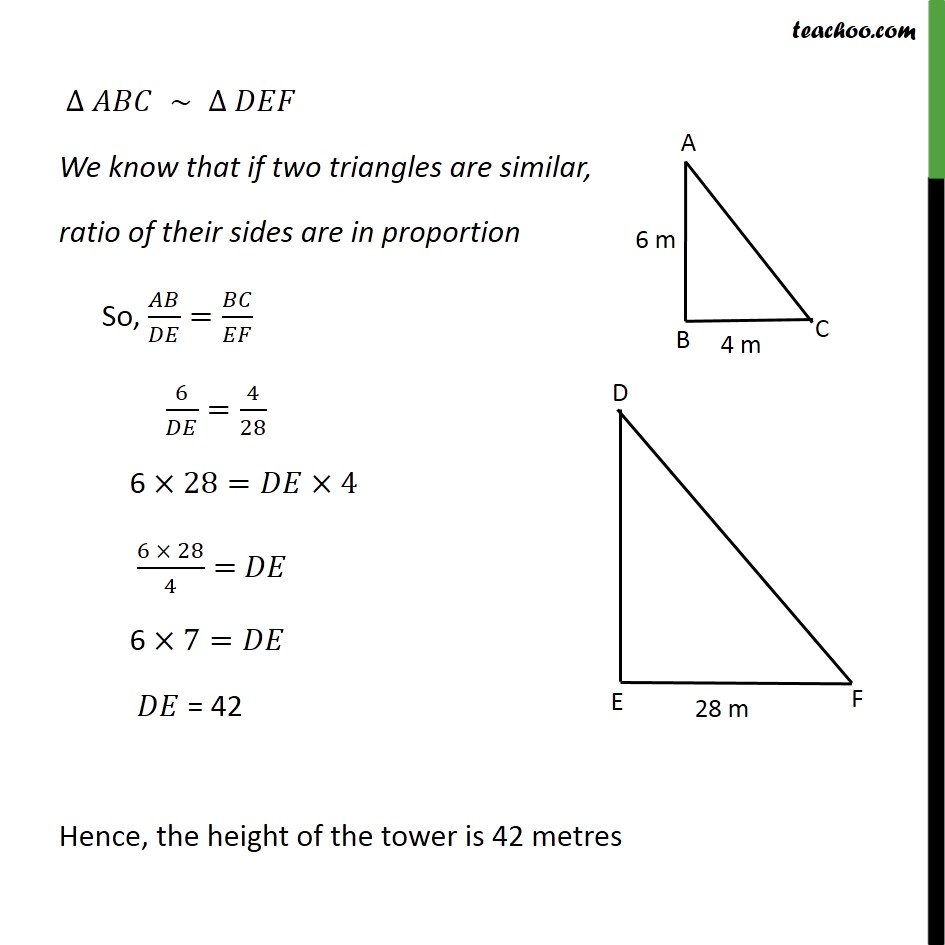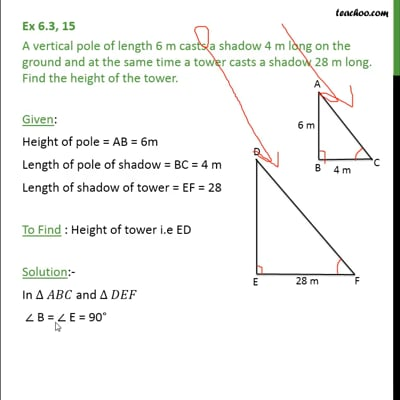Ex 6.3

Chapter 6 Class 10 Triangles
Serial order wiseThis video is only available for Teachoo black users

Solve all your doubts with Teachoo Black (new monthly pack available now!)

### Transcript

Ex 6.3, 15 A vertical pole of length 6 m casts a shadow 4 m long on the ground and at the same time a tower casts a shadow 28 m long. Find the height of the tower. Given: Height of pole = AB = 6m Length of pole of shadow = BC = 4 m Length of shadow of tower = EF = 28 To Find : Height of tower i.e ED Solution:- In ∆ 𝐴𝐵𝐶 and ∆ 𝐷𝐸𝐹 ∠ B = ∠ E = 90° ∠𝐶=∠𝐹 ∴ ∆ 𝐴𝐵𝐶 ∼ ∆ 𝐷𝐸𝐹 ∆ 𝐴𝐵𝐶 ∼ ∆ 𝐷𝐸𝐹 We know that if two triangles are similar, ratio of their sides are in proportion So, 𝐴𝐵/𝐷𝐸=𝐵𝐶/𝐸𝐹 6/𝐷𝐸=4/28 6 ×28=𝐷𝐸×4 (6 × 28)/4= 𝐷𝐸 6 ×7=𝐷𝐸 𝐷𝐸 = 42 Hence, the height of the tower is 42 metres